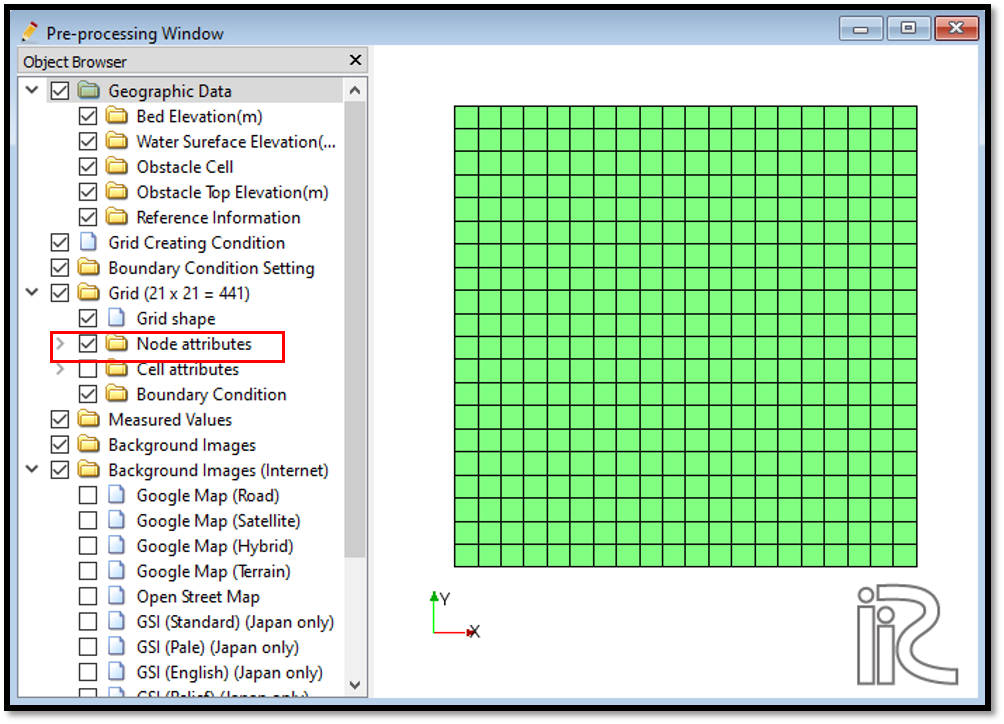# Example 02: Upward flow in a tank

## Purpose

To calculate the density currents in an upward flow originated from a tank bottom. A low density boundary which has a lesser density than the background density generates this flow in a tank.

## Creation of calculation grid and setting initial conditions

Create the calculation grid using [Grid] [Select Algorithm to Create Grid]. Then select Grid Creation Algorithem window will appear and then select [Gird Generator for Nays3DV] in select grid creating algorithm window.

Then grid creation window will appear. Select channel shape as shown in Figure 32.

Then we can give channel bed condition. As here we use the default condition flat(no bar) no modifications are needed.

If new grids are added or width is varied it is possible to set them. As in this example no grids added and no width variations, no modifications are needed in them.

Initial water surface profile tab is used to give downstream depth, water surface slope and initial water surface purtavation. It can be seen as shown in Figure 33.

Here a tank is selected with a flat bed. After giving all the parameters as given in figures, select [Create grid]. Here a confirmation dialogue box will appear to map geographic data to grid attributes. Select yes and it will map the geographic data to the created grid.

The grid will be created as shown in Figure 34.

Then by selecting node attributes and bed elevation in object browser, [Object Browser] - [Grid] - [Node attributes] - [Bed Elevation (m)], it is possible to see the geographic data mapped grid as shown in Figure 35.Figure 35 : Grid creation : Created Grid after mapping geographic data

It is always safer to see the attributes after mapping.

Now save the project with [File] [Save project as .ipro].

## Setting the calculation conditions and simulation

Give the calculation conditions with, [Calculation Condition] [Settings].

Calculation cndition window will appear. Give computational parameters as shown in Figure 36.

Now give the [Hydraulic Boundary Conditions]. Since the boundary conditions for this simulation were given as closed boundaries in computational parameters, hydraulic boundary conditions window is inactive in this example as shown in Figure 37.

Now give [Initial and Boundary Concentration] as shown in Figure 38.

Here background concentration is the concentration in the tank and purturbed concentration is concentration of the down object which we create. In an upflow it is necessary to give initial purturbed concentration a lower value than the background concentration. Otherwise the upward flow won’t occur.

In the initial concentration distribution it is necessary to select the area of new concentration in all the directions i, j, k start and end grids.

Give time and iteration parameters as shown in Figure 39.

Give physical parameters as shown in Figure 40.

After setting the calculation conditions, save the project by clicking on save tab. Now start simulation by, [Simulation] [Run]. Simulation will start and after some time it will finish showing the message the solver finished the calculation.

## Visualization of results

Open 3D post processing window by selecting, [Calculation Results] [Open new 3D Post-Processing Window].

Select any parameter in [Object Browser], [iRIC Zone].

In this example isosurface is selected as shown in Figure 41. When [Object Browser] - [Isosurface] - [Add] is selected, we can add isosurface of any parameter such as concentration, pressure, position, eddy viscosity, velocity etc. In this example concentration is selected.

In isosurface setting window, it is necessary to set physical value such as concentration, pressure, velosity etc which we need to plot. In value setting , we can see min value and max value as shown in Figure 42. Depending on our requirement we can select a value in between that min and max value.

Then we can select the colour for the isosurface as shown in Figure 43.

Then we can see the isosurface of concentration as shown in Figure 44.

Initial and final isosurfaces can be seen as shown in Figure 45.